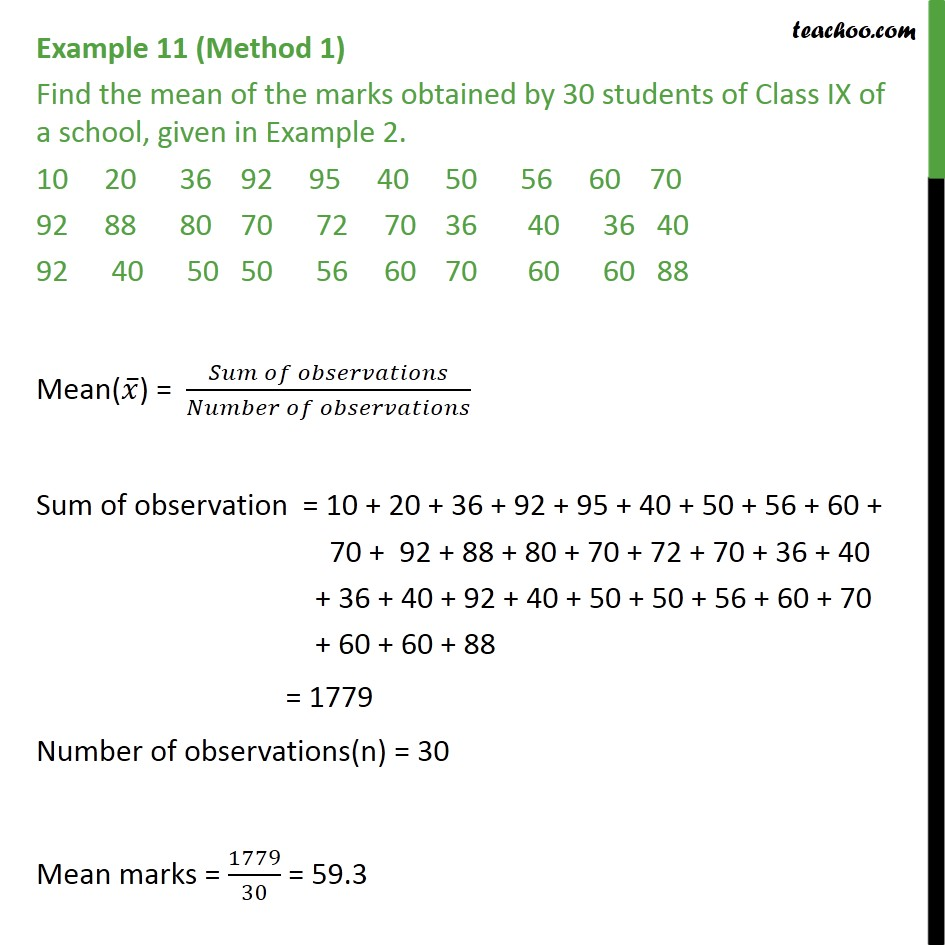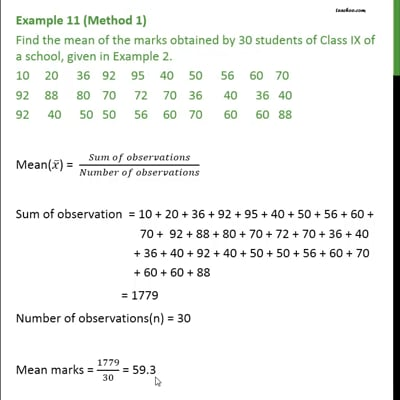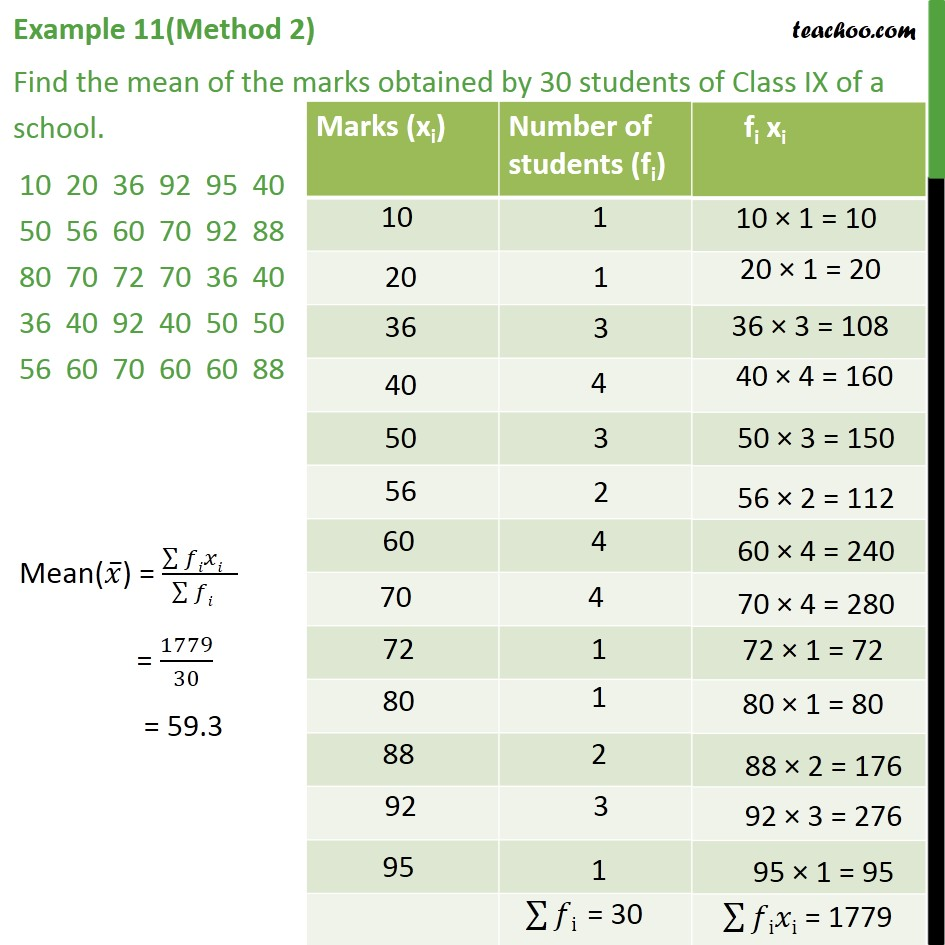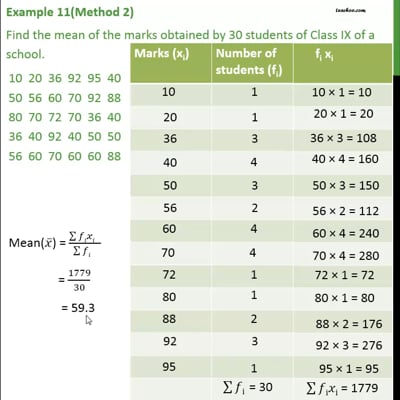Mean

Chapter 14 Class 9 Statistics
Concept wiseThis video is only available for Teachoo black usersThis video is only available for Teachoo black users

Solve all your doubts with Teachoo Black (new monthly pack available now!)

### Transcript

Example 11 (Method 1) Find the mean of the marks obtained by 30 students of Class IX of a school, given in Example 2. 10 20 36 92 95 40 50 56 60 70 92 88 80 70 72 70 36 40 36 40 92 40 50 50 56 60 70 60 60 88 Mean(𝑥 ̅) = (𝑆𝑢𝑚 𝑜𝑓 𝑜𝑏𝑠𝑒𝑟𝑣𝑎𝑡𝑖𝑜𝑛𝑠)/(𝑁𝑢𝑚𝑏𝑒𝑟 𝑜𝑓 𝑜𝑏𝑠𝑒𝑟𝑣𝑎𝑡𝑖𝑜𝑛𝑠) Sum of observation = 10 + 20 + 36 + 92 + 95 + 40 + 50 + 56 + 60 + 70 + 92 + 88 + 80 + 70 + 72 + 70 + 36 + 40 + 36 + 40 + 92 + 40 + 50 + 50 + 56 + 60 + 70 + 60 + 60 + 88 = 1779 Number of observations(n) = 30 Mean marks = 1779/30 = 59.3